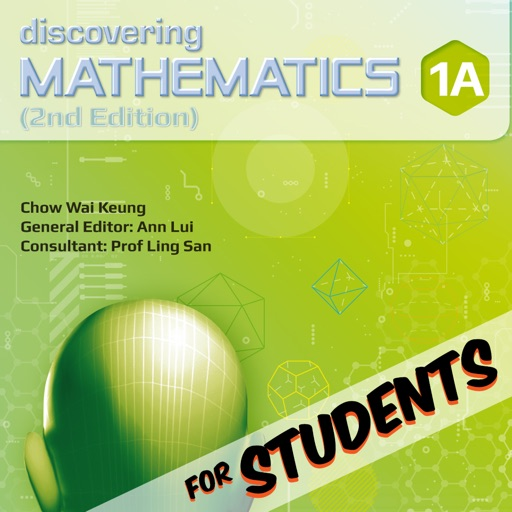Learn mathematics on the go and ace your math exams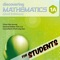Discovering Mathematics 1A (Express) for Students

by Star Publishing Pte Ltd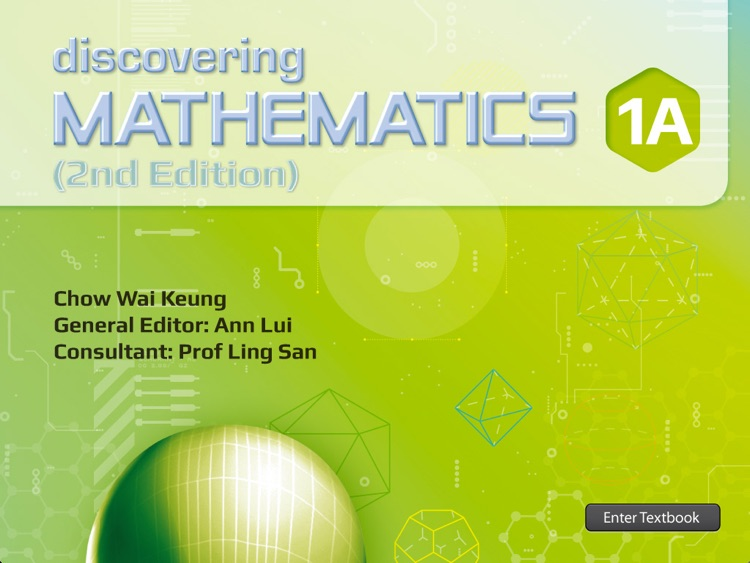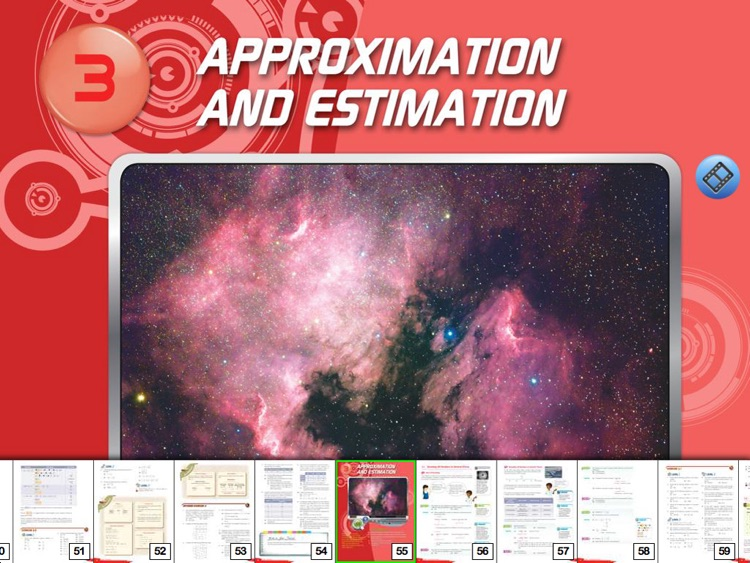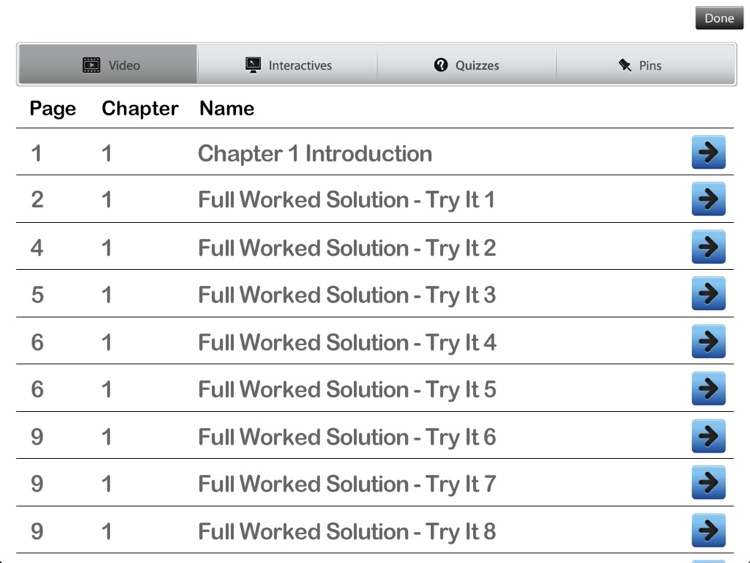Learn mathematics on the go and ace your math exams!Version
2.0.2
Rating
NA
Size
335Mb
Genre
Education Books
Last updated
March 1, 2017
Release date
January 13, 2015

App Screenshots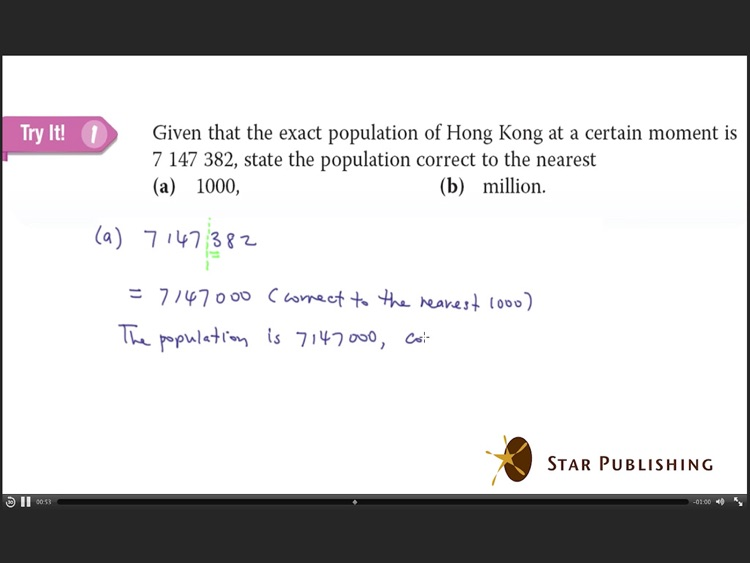App Store Description

Learn mathematics on the go and ace your math exams!
Discovering Mathematics 1A Express (digital format) is part of the comprehensive package of the best-selling Discovering Mathematics (2nd edition) textbook series, approved for use by MOE, Singapore.
This digital book includes the textbook, video, worked solution and step-by-step video guide for solving problems.
Give yourself the winning edge to excel in maths now!
Interactive Features:
• Textbook (extraction/zooming functions)
• Animated videos as chapter openers
• Step-by-step video guide on solving problems
• Embedded calculator
• NEW Interactive Whiteboard
• Pin-a-note function
• Drawing pen for user to write freely on any part of the book
Contents
• Factors and Multiples
• Real Numbers
• Approximation and Estimation
• Introduction to Algebra
• Algebraic Manipulation
• Simple Equations in One Variable
• Angles and Parallel Lines
• Triangles and Polygons
• Additional guide to Problem-solving Processes and Heuristic